# Python DateTime

Problem Statement: To understand Python DateTime.

In python, anything that has to do with date and time is handled by the datetime module, which further categorizes the module into different classes. Or we can say that a python datetime is a module that supplies classes to work with date and time. These classes provide functions to deal with dates, times, and time intervals.

Classes of datetime module :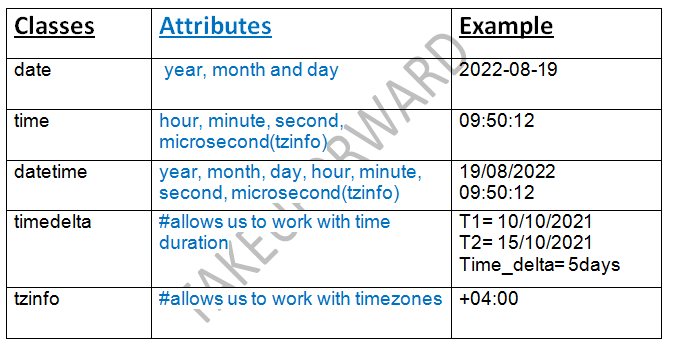The list of standard libraries we can use to work with date and time.

1. datetime: The datetime is a built-in module that provides functions to handle many complex functionalities involving the date and time. It has a date, time, timezone, and timedelta classes to work with date and time.
2. calendar: In Python, a calendar is a built-in module to work with calendar-related activities and operations. In the calendar module, by default, Monday is the first day of the week, and Sunday is the last day of the week as per the Gregorian calendar (the calendar introduced in 1582 by Pope Gregory XIlI).
3. time: time module provides a different function to work with time-related activities where dates are not needed.

Let’s see some more details about classes in datetime :

date Class in DateTime

We can represent the date object using the date class and the date class initializes the native date. It always considers the Gregorian calendar ( format YYYY-MM-DD). The constructor of the date class takes three parameters which include year, month, and day.

Syntax:

`datetime.date(year, month, day)`

time Class:

To work with time, we can use the time class from the datetime module. It is independent of day.

Syntax:

```datetime.time(hour, minute, second, microsecond, tzinfo, *, fold)
```

datetime Class

The datetime class contains information about both date and time. It Assumes the Gregorian Calendar and there are 86400 seconds every day.

Syntax:

`datetime.datetime(year, month, day, hour, minute=0, second, microsecond, tzinfo=None)`

TimeDelta:

A timedelta represents a duration which is the difference between two dates, time, or datetime instances, to the microsecond resolution.

Using the datetime module’s timedelta class we can add or subtract weeks, days, hours, minutes, seconds, microseconds, and milliseconds from a given date and time.

Syntax:

```class datetime.timedelta(days=0, seconds=0, microseconds=0, milliseconds=0, minutes=0, hours=0, weeks=0)
```

Returns: Date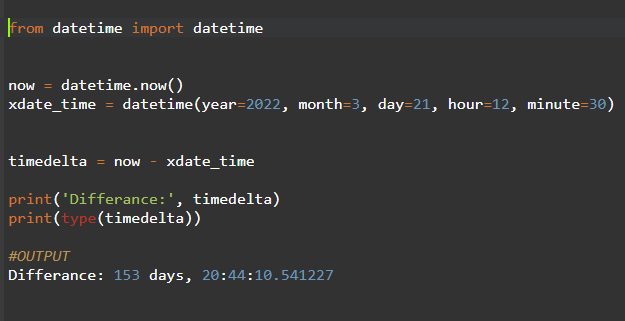## Python DateTime Formatting

DateTime to String:

We can represent date and time in various formats in Python using the strftime() function of a datetime module and time module. The strftime() method returns a string representing a datetime object according to the format codes.

String to DateTime:

We can parse a string to a datetime object using the strptime() with the various standard date formatting codes available in Python.

Now lets us see some datetime format codes: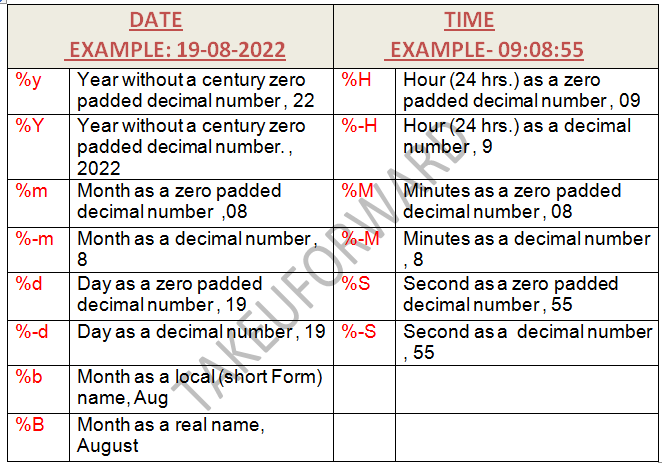Python Time module

Python Time module provides various functions to perform time-related things/activities.

Lets us now see some of the function of this module.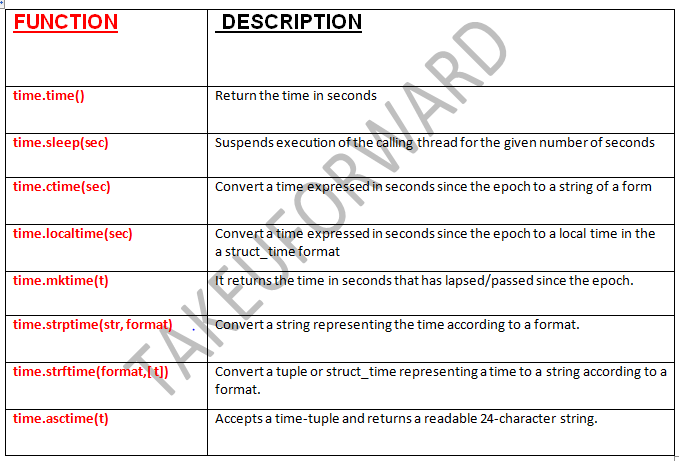## Python Calendar Module

Python has a built-in module called calendar for calendar-related activities. This module supplies calendar-related functions, including functions to print a text calendar for a given month or year. By default, the calendar takes Monday as the first day of the week and Sunday as the last one. To use the calendar first, we need to Import it.

Let’s see some of the functions in the calendar module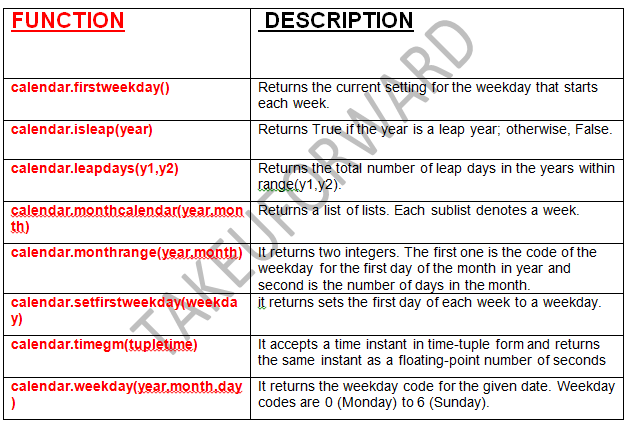Example1:

Let’s code a simple problem where we need to display the current time with all the components to display the date, time, year, month, day, hours, minutes, seconds, and microseconds of the current date.

Code:

## Python Code

``````from datetime import datetime

dt = datetime.now()
current_date = datetime.now()

print("Datetime is:", dt)

print('Date:', dt.date())
print('Time:', dt.time())
print('Timestamp:', dt.timestamp())

print('Year:', dt.year)
print('Month:', dt.month)
print('Day:', dt.day)

print('Hours:', dt.hour)
print('Minutes:', dt.minute)
print('Seconds:', dt.second)
print('Microseconds:', dt.microsecond)
``````

Output:

Datetime is: 2022-08-23 15:42:04.230777
Date: 2022-08-23
Time: 15:42:04.230777
Timestamp: 1661269324.230777
Year: 2022
Month: 8
Day: 23
Hours: 15
Minutes: 42
Seconds: 4
Microseconds: 230777

Example2:

Let’s code a program where we had to display the calendar for 2022 (august month). And we will import time to display the current time in seconds, local time, time in string form, Formatting the time to display in string format, and String to Time Object by using the time and calendar module.

Code:

## Python Code

``````import calendar
import time

year = 2022
month = 8

print(calendar.month(year, month))

seconds = time.time()

print("Current time in seconds:", seconds)

print("\n\n")

local_time = time.localtime(seconds)
print('Local Time', local_time)
print("\n\n")

print('Time in String:', time.ctime(seconds))
print("\n\n")

print('Formatted Time:', time.strftime("%d/%m/%Y %H:%M:%S", local_time))
print("\n\n")

print('Time object:', time.strptime("22/08/2022 08:38:37", "%d/%m/%Y %H:%M:%S"))
``````

Output:

``August 2022``

Mo Tu We Th Fr Sa Su
1 2 3 4 5 6 7
8 9 10 11 12 13 14
15 16 17 18 19 20 21
22 23 24 25 26 27 28
29 30 31

Current time in seconds: 1661269404.9738889

Local Time time.struct_time(tm_year=2022, tm_mon=8, tm_mday=23, tm_hour=15, tm_min=43, tm_sec=24, tm_wday=1, tm_yday=235, tm_isdst=0)

Time in String: Tue Aug 23 15:43:24 2022

Formatted Time: 23/08/2022 15:43:24

Time object: time.struct_time(tm_year=2022, tm_mon=8, tm_mday=22, tm_hour=8, tm_min=38, tm_sec=37, tm_wday=0, tm_yday=234, tm_isdst=-1)

Special thanks to AYUSH KUMAR for contributing to this article on takeUforward. If you also wish to share your knowledge with the takeUforward fam, please check out this articleIf you want to suggest any improvement/correction in this article please mail us at [email protected]